The Extended ScoreCard Outlier Analysis (Adjusted)

The below Metrics don't appear to be converted to %.

TotalOutliersPct
PositiveOutliersPct
NegativeOutliersPct
OutlierProfitPct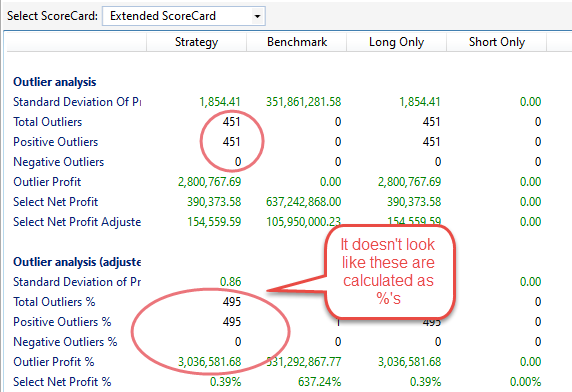The displayed values could be helpful for some analysis. I'd like to suggest correcting the calculations for the above, but also adding:

1
209
Solved

Bookmark

Sort
Actually there's no bug, everything is correct. These metrics are not a percentage of outlier trades. It's a count of outlier trades based on percentages rather than dollars as in the original formula. You can read more on this here:

0
Let's make it look like this in PowerPack B12: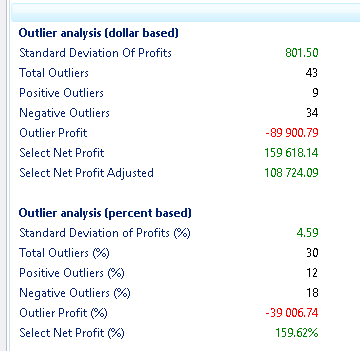0
Thank you Eugene for the reference post. I beleive I have a better understanding of the intent behind these metrics and how they are calculated.

However, I'm still left questioning the values. I've tried exporting the trade list and calculating them myself (as I understand them to be) but I fail to reach the same results.

The term Outlier is defined in the referenced post and the Metric Tip as a trade which has a profit at or beyond 3 * Stdev +/- from the mean of profits (I assume profits includes negative profits).

As I understand it,

1) The original values (based on \$) are calculated using \$ profits made per trade, and:

2) The % values are generated by using the %profit per trade data in an atempt to avoid a skewing of the mean due to increase in \$ allocation and therefore relative profit (+/-) as equity grows (especially with a % of Profit position size rule employed)

3) Both methods utilize the same functions for calculating results, only the data fed in is chaged from \$profit to %profit.

I can understand how the metrics might be helpful, however in the example below all 495 trades produced by the strategy are classified as outliers when using the % based aproach.

This seems odd to me. Generally speaking, in a normalized distribution one would expect less than 0.5% of the data to be considered an outlier acording to the definition above. I understand that the very nature of trading system development, if successful, is biased toward profitability more than loss, therefore a skewed distribution is more likely (Hopefully). One would expect that the further the skew is to the left, the more profitable the system.

In the case below, this strategy had a 100% win rate with profit target at 8.25% and % based position sizing over 25years. Somehow, it has resulted in ALL trades identified as outliers.

Of the 495 trades produced 478 produced profits between 8.25 and 9.76. The remaining are scattered between 10% and 17.76% .The Standard deviation is 0.86. Even if the mean of %profits sat at 9.76% the vast majoirty of trades would fit into 2*Stdev from the mean. (9.76 - (2*0.86)) = 8.24

Are you able to show the code snippet that calculates these metrics so I can better understand there calculation? If not, that's ok. Eitherway, I appreciate the feedback and article reference.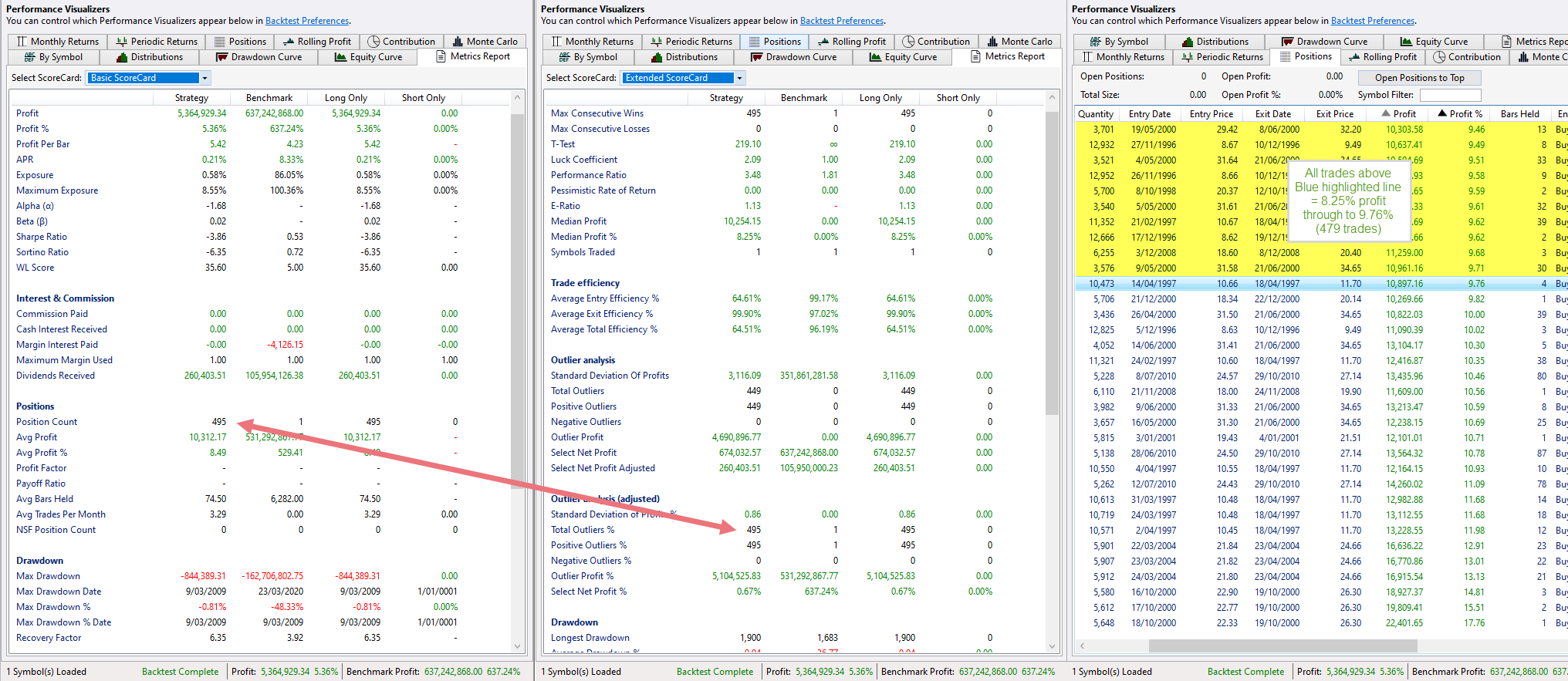1
Thanks for the follow-up. Could you e-mail the lists of Profit and Profit % to support@wealth-lab.com? Right click the Positions tab and choose "Copy to clipboard".

Also for Select Net Profit %, what is the starting capital?
0
Eugene, it looks like the Percentage metric (the second one circled) is still reporting raw numbers and not percent ...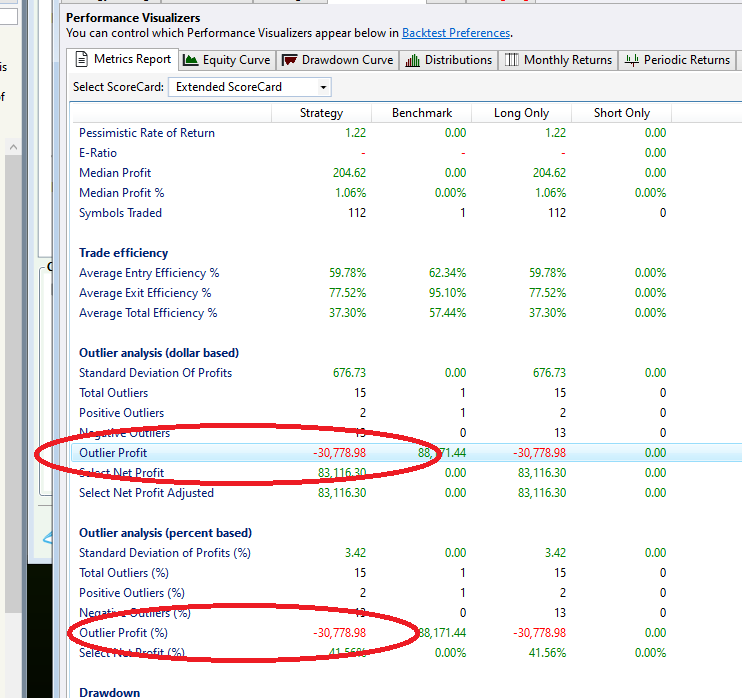0
QUOTE:
Eugene, it looks like the Percentage metric (the second one circled) is still reporting raw numbers and not percent ...

Dion, this is neither a percentage figure. Same absolute value, just an adjusted calculation. There are none in both groups. So adding the "(%)" suffix (as on our screenshots) is still confusing. :( But leaving them both synonymous would also be very ambiguous either?
0
QUOTE:
But leaving them both synonymous would also be very ambiguous either?

Probably not. Let's make it the way it's been in WL6 then! Will also document this in the help.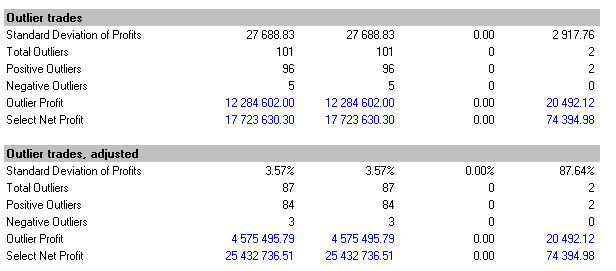0
Eugene, look at the values:

Outlier Profit = -30,778,98
Select Net Profit = 83,116.30
Select Net Profit Adjusted = 81,116.30

Outlier Profit % = -30,778.98 (same as Outlier Profit)
Select Net Profit % = 41.56%

It just looks to me like a copy/paste error and you're not sending the correct value to the metrics report for Outlier Profit %.

I figure this because, while Select Net Profit clearly changed in the percent version, Outlier Profit did not. Can you check?
0
QUOTE:
Select Net Profit % = 41.56%

Ouch, it shouldn't be a percent. Good catch and glad this hasn't leaked to production build! :)

```CODE://wrong (PowerPack beta):
reportHost.AddMetricDouble("SelectNetProfitPct", true, true, 2, "Select Net Profit");
//right as seen before:
reportHost.AddMetricDouble("SelectNetProfitPct", true, false, 2, "Select Net Profit");```

0
Why is Outlier Profit and Outlier Profit % the same value? Can you explain them in plain English?
0
There is no "Outlier Profit %" anymore in my latest commit. Both group of values are absolute numbers. It's that the second group's numbers internally are based on percentage figure and the "classic" group is dollar-based.

Simply put, the first group is based on Position.Profit and the second group derives from Position.ProfitPercent. But the logic remains the same.
1
Thanks for taking a closer look at this Eugene.

Positions.txt and Stategy settings screen shot sent to support.
0
Hi Daniel,

Got your files. I spotted an unfortunate bug in the code which renders the number of outliers and select net profit incorrect for both groups. A fix is coming ASAP in PowerPack build 12. Thanks for staying alert and sorry for the inconvenience!
0
I thought that might be the case.... :-)

Thanks again for taking a closer look.
0
Eugene, while you're in the code, take a look at the Standard deviation in \$ amount. I can't duplicate that either. Not in excel or in code.

If you dump the positions list into excel and use the STDEV function on the \$ profit column i think you will find it comes to \$1,191.39

That's a long way short of the \$3,116.09 reported on the Extended Score Card

Are you using the Math.Numerics StDev functions, or did you write your own? I wrote my own today, and it matches Excel. However excel and my code do come to the same value of 0.86 forthe Stdev(%profits-per-position), which might indicate the data for the \$ pass is not the \$profit-per-position but something else.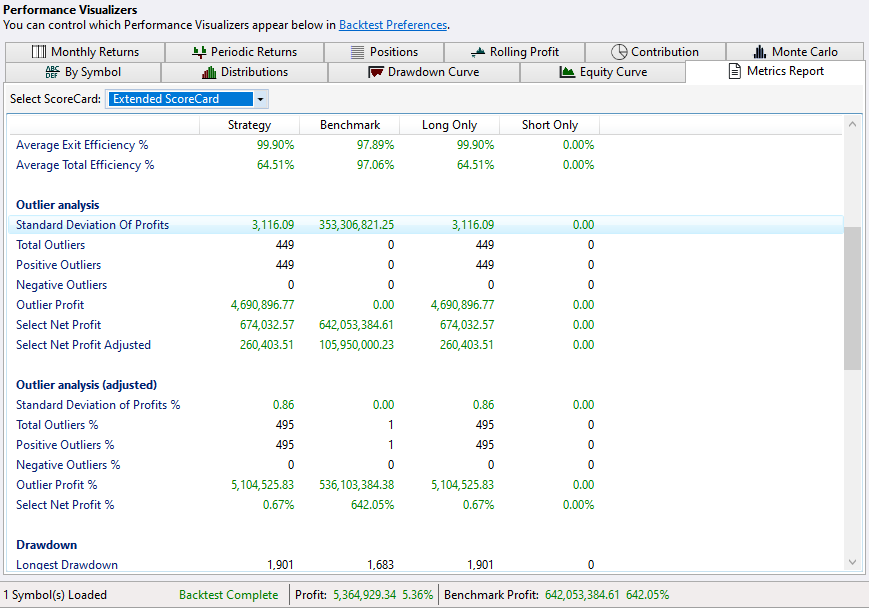1
I'm using own code. After fixing it for PowerPack B12 I ran it against your profit values from the file and the standard deviation came out identical with your numbers (i.e. as ~\$1191 / 0.86).
1